# Electronics and Communication Engineering - Analog Electronics

41.

In a self bias circuit for CE amplifier, an increase in emitter resistance RE results in

 A. increase in emitter voltage B. decrease in emitter voltage C. increase in emitter current D. decrease in emitter current

Explanation:

Emitter voltage is the same. Increase of emitter resistance decreases with emitter current.

42.

For a BJT if β = 50, ICEO = 3 μA and IC = 1.2 mA then IE

 A. 1 mA B. 1.2 mA C. 1.3 mA D. 1.4 mA

Explanation:

IE = IC + IB .

43.

In the CE circuit shown in figure the slope of ac load line will be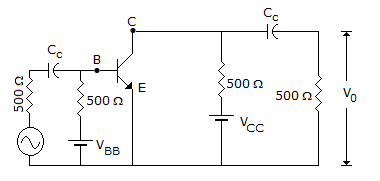A.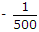B.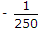C.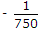D.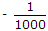Explanation: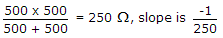.

44.

When the circuit is switched on, the loop gain of a Wien bridge oscillator is

 A. 1 B. more than 1 C. less than 1 D. about 0.5

Explanation:

The initial loop gain must be more than 1.

45.

The op-amp circuit shown in the figure is a filter. The type of filter and its cut off type of filter and its cut off frequency are respectively.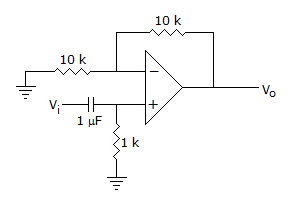Explanation:

At 1000 Hz frequency,

Hence, high pass filter,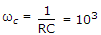.